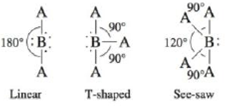# There are several molecular structures based on the trigonal bipyrarnid geometry (see Table 4-3). Three such structures are Which of the compounds/ions Br 3 − , ClF 3 , XeF 4 , SF 4 , PF 5 , ClF 5 , and SF 6 have these molecular structures?### Chemistry: An Atoms First Approach

2nd Edition
Steven S. Zumdahl + 1 other
Publisher: Cengage Learning
ISBN: 9781305079243

#### Solutions

Chapter
Section### Chemistry: An Atoms First Approach

2nd Edition
Steven S. Zumdahl + 1 other
Publisher: Cengage Learning
ISBN: 9781305079243
Chapter 4, Problem 25E
Textbook Problem
1 views

## There are several molecular structures based on the trigonal bipyrarnid geometry (see Table 4-3). Three such structures areWhich of the compounds/ions Br3−, ClF3, XeF4, SF4, PF5, ClF5, and SF6 have these molecular structures?

Interpretation Introduction

Interpretation: The molecular structure of given compound is to be predicted from given structures.

Concept introduction: Molecular structure is a three-dimensional shape of a molecule.

Bond angle is the angle between atoms of molecule.

To determine: The correct molecular structure of Br3,ClF3,XeF4,SF4,PF5,ClF5 and SF4 (including bond angles) according to given structure.

### Explanation of Solution

The steps are to be followed to determine the molecular structure is:

• Identify the central atom.
• Count its valence electrons.
• Add or subtract electrons for charge.
• Draw the Lewis structure.
• Divide the total number of electrons by 2 to find the number of electron pairs.
• Use this number to predict the shape.

The atomic number of bromine (Br) is 35 and its electronic configuration is,

1s22s22p63s23p63d104s24p5

The valence electron of bromine is 7

The total number of valence electrons is,

3Br+1e=7×3+1=22

The Lewis structure of Br3 is

Figure 1

The formula of number of electron pairs is

X=V+M±C2

Where,

• X is number of electron pairs
• V is valence electrons of central atom
• M is number of monovalent atoms
• C is charge on compound

The number of electron pairs is

X=V+M±C2X=7+12=4

The shape of compound is linear and bond angle is 1800

Explanation:

The steps are to be followed to determine the molecular structure is:

• Identify the central atom.
• Count its valence electrons.
• Add or subtract electrons for charge.
• Draw the Lewis structure.
• Divide the total number of electrons by 2 to find the number of electron pairs.
• Use this number to predict the shape.

The atomic number of (central atom) chlorine (Cl) is 17 and its electronic configuration is,

1s22s22p63s23p5

The valence electron of chlorine is 7

The atomic number of fluorine (F) is 9 and its electronic configuration is,

1s22s22p5

The valence electron of fluorine is 7

The total number of valence electrons is,

3Cl+F=7×3+7=28

The Lewis structure of ClF3 is

Figure 2

The formula of number of electron pairs is

X=V+M±C2

Where,

• X is number of electron pairs
• V is valence electrons of central atom
• M is number of monovalent atoms
• C is charge on compound

The number of electron pairs is

X=V+M±C2X=7+32=5

The shape of compound is T-shape.

Explanation:

The steps are to be followed to determine the molecular structure is:

• Identify the central atom.
• Count its valence electrons.
• Add or subtract electrons for charge.
• Draw the Lewis structure.
• Divide the total number of electrons by 2 to find the number of electron pairs.
• Use this number to predict the shape.

The atomic number of central atom sulfur (S) is 16 and its electronic configuration is,

1s22s22p63s23p4

The valence electron of sulfur is 6

The atomic number of fluorine (F) is 9 and its electronic configuration is,

1s22s22p5

The valence electron of fluorine is 7

The total number of valence electrons is,

S+4F=6+4×7=34

The Lewis structure of SF4 is

Figure 3

The formula of number of electron pairs is

X=V+M±C2

Where,

• X is number of electron pairs
• V is valence electrons of central atom
• M is number of monovalent atoms
• C is charge on compound

The number of electron pairs is

X=V+M±C2X=6+42=5

The shape of compound is see-saw-shape.

Explanation:

The steps are to be followed to determine the molecular structure is:

• Identify the central atom.
• Count its valence electrons.
• Add or subtract electrons for charge.
• Draw the Lewis structure.
• Divide the total number of electrons by 2 to find the number of electron pairs.
• Use this number to predict the shape

### Still sussing out bartleby?

Check out a sample textbook solution.

See a sample solution

#### The Solution to Your Study Problems

Bartleby provides explanations to thousands of textbook problems written by our experts, many with advanced degrees!

Get Started

Find more solutions based on key concepts
Explain what is meant by the term balanced chemical equation.

General Chemistry - Standalone book (MindTap Course List)

What is a redox reaction?

Biology: The Unity and Diversity of Life (MindTap Course List)

A way to prevent spoilage of unsaturated fats and make them harder is to change their fatty acids chemically th...

Nutrition: Concepts and Controversies - Standalone book (MindTap Course List)

2-72 (Chemical Connections 2D) Bronze is an alloy of which two metals?

Introduction to General, Organic and Biochemistry

How can astronomers detect neutrinos from the sun?

Horizons: Exploring the Universe (MindTap Course List)

Hookes law describes a certain light spring of unstretched length 35.0 cm. When one end is attached to the top ...

Physics for Scientists and Engineers, Technology Update (No access codes included)

Is Earths magnetic field a constant? That is, would a compass needle always point north?

Oceanography: An Invitation To Marine Science, Loose-leaf Versin